# Catch up on the latest AI articles# Advanced Offline Model-based Reinforcement Learning!　Solving A Task With A Real Robot From Image Data?

3 main points
✔️ We propose LOMPO, a new Offline Model-based RL methodology
✔️ on the latent space
Uncertainty quantification on the latent space
✔️ We were able to solve the task for real-world robots

written by Rafael RafailovTianhe TuAravind RajeswaranChelsea Finn
(Submitted on 21 Dec 2020)

Subjects: Machine Learning (cs.LG); Artificial Intelligence (cs.AI); Robotics (cs.RO)

## first of all

In recent years, online RL (reinforcement learning) as well as offline RL has been attracting attention due to its various advantages. However, it is important to learn policies using images as input for real-world robots, not for simulations. Therefore, in this article, we introduce a method called offline model-based policy optimization (LOMPO) that can learn policies even when images are used as input. Because this method is model-based, it obtains a policy by modeling the transitions in the environment and then performing policy optimization based on the modeling. The figure below shows the overall flow of the method introduced in this article. Since it is an offline RL, the task data is given in advance. Then, the policy is learned by the proposed method, LOMPO, and finally the performance is evaluated using a robot.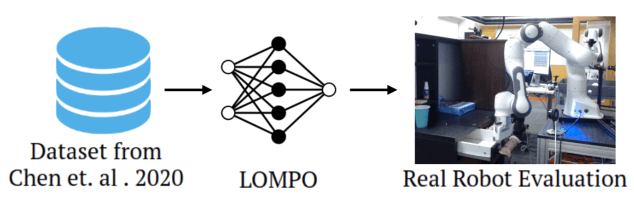So why is off-line model-based RL so difficult, especially when the input is an image? So far, offline model-based RL has been used to quantify the uncertainty of the observations generated by the trained model (e.g., by taking the variance of the prediction), and to penalize the observations when the uncertainty is large, in order to avoid extrapolation error and errors due to unknown observations (error on out-of-distribution). However, this method is inefficient and unsuitable for images because of the high dimensionality of the generated images. Therefore, the proposed method, LOMPO, learns the dynamics in the latent space and quantifies the uncertainty in the latent space, so that it can learn the policy even when the input is an image. Now, I will introduce the detailed method.

## technique

The ultimate goal is to obtain an offline model-based RL that can handle images as high-dimensional inputs. As mentioned above, previous offline model-based RLs have quantified the uncertainty of the generated observations, but it is very costly and impractical to generate images and quantify the uncertainty. Therefore, the proposed method, LOMPO, learns a latent dnamics model (a model of dynamics on a latent space) and quantifies the uncertainty by ensemble on the latent space. In other words, LOMPO learns dynamics in low-dimensional space, not in high-dimensional space such as images. First of all, the MDP (Markov Decision Process), which is the original problem, is defined as uncertainty-penalized latent MDP, which is MDP with uncertainty penalty added to reward, and then, since the input is an image The uncertainty-penalized POMDP (Partially Observable MDP) is made, and the policy and the latent dyanmics model are optimized on it. I will explain this flow in detail in the following.

### On the Quantification of Model Uncertainty on Latent Spaces

In order to quantify the uncertainty on the latent space, we will organize around the MDP. First, we define the MDP on the latent space $\mathcal{S}$ as $M_{\mathcal{S}}=(\mathcal{S}, \mathcal{A}, \mathcal{T}, r, \mu_{0}, \gamma)$, and similarly, we define the estimated MDP on the latent space as $\widehat{ M}_{\mathcal{S}} = (\mathcal{S}, \mathcal{A}, \widehat{\mathcal{T}}, r, \mu_{0}, \gamma)$. Let $\widehat{T}(s'|s, a)$ be a latent dynamics model. The ultimate goal of the proposed method is to find $\widehat{M}_{\mathcal{S}}$. in in $\widehat{M}_{\mathcal{S}}$, and at the same time maximize the reward in $M_{\mathcal{S}}$. To do so, we first learn $M_{\mathcal{S}}$ and $\widehat{M}_{\mathcal{s}}$. to create an uncertainty-penalized MDP $\widetilde{M}_{\mathcal{S}}=(\mathcal{S}, \mathcal{A}, \widehat{T}, \tilde{r}, \mu_{0}, \gamma)$. Here $\tilde{r}$ is represented as $\tilde{r}(s, a)-\lambda u(s,a)$, where $u(s, a)$ is the uncertainty estimator. Then, the return of policy $\pi$ in uncertainty-penalized MDP is the lower bound of the return in real MDP, and the difference of the return depends on the error of latent dynamic model. If you are interested in the detailed definition, please refer to the paper. In this paper, we use this uncertainty-penalized MDP to train the model and optimize the policy.

### On the optimization of Latent Model and Policy using Uncertainty-penalized ELBO

In this section, we introduce the optimization of the latent dynamics model and policy based on the uncertainty-penalized MDP introduced in the previous section. First of all, based on the uncertainty-penalized MDP, the uncertainty-penalized POMDP $\widehat{M} = (\mathcal{X}, \mathcal{S}, \mathcal{A}, \widehat{T}, D, \ tilde{r}, \mu_{0}, \gamma)$ is defined. Here, $\mathcal{X}$ represents the image space. dynamic $\widehat{T}(s_{t+1}|s_{t}, a_{t})$, and policy $\pi(a_{t}|x_{1:t}, a_{1:t-1})$ as follows.

$$\widehat{q}\left(s_{1: H}, a_{t+1: H} \mid x_{1: t+1}, a_{1: t}\right)=\prod_{\tau=0}^{t} q\left(s_{\tau+1} \mid x_{\tau+1}, s_{\tau}, a_{\tau}\right) \prod_{\tau=t+1}^{H-1} \widehat{T}\left(s_{\tau+1} \mid s_{\tau}, a_{\tau}\right) \prod_{\tau=t+1}^{H} \pi\left(a_{\tau} \mid x_{1: \tau}, a_{1: \tau-1}\right)$$

And from this $\widehat{q}$ we can express the expected value of the reward as follows.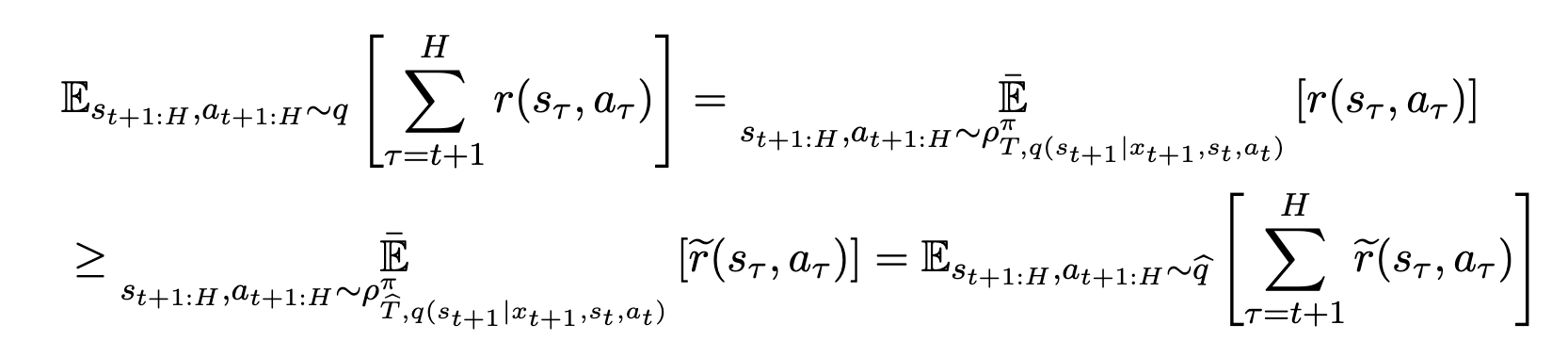For a detailed introduction, please refer to the paper. What is particularly important here is that the expected value of reward under uncertainty-penalized MDP is expressed as the lower-bound of the expected value of reward under true MDP. Then, using this formula, we can express the below formula (see), which is the ELBO (Evidence Lower Bound) under the normal POMDP, as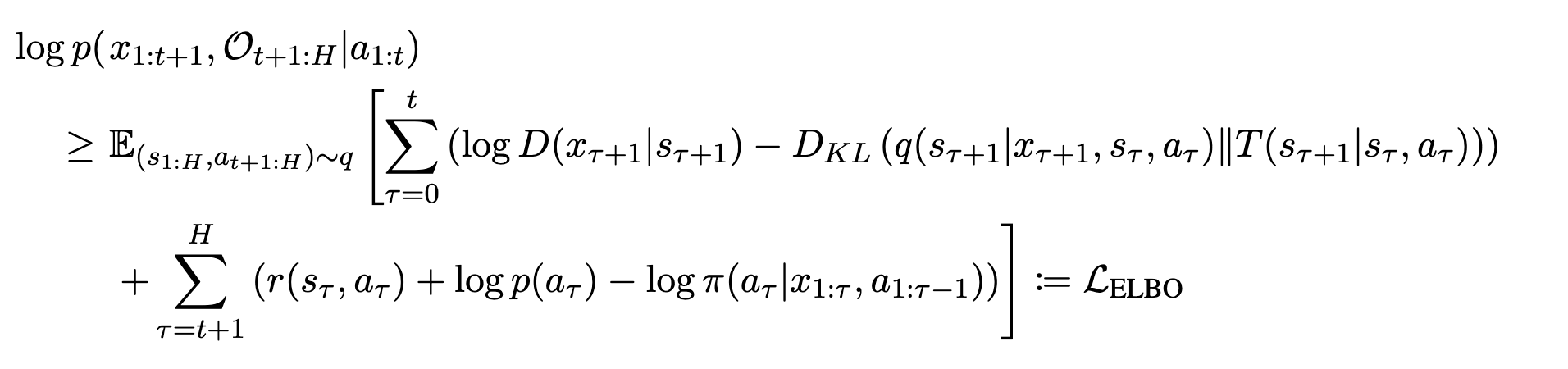As shown below, it can be made into an equation relating $\widetilde{\mathcal{L}}_{ELBO}$ and $\mathcal{L}_{ELBO}$, which is the ELBO in uncertainty-penalized MDP.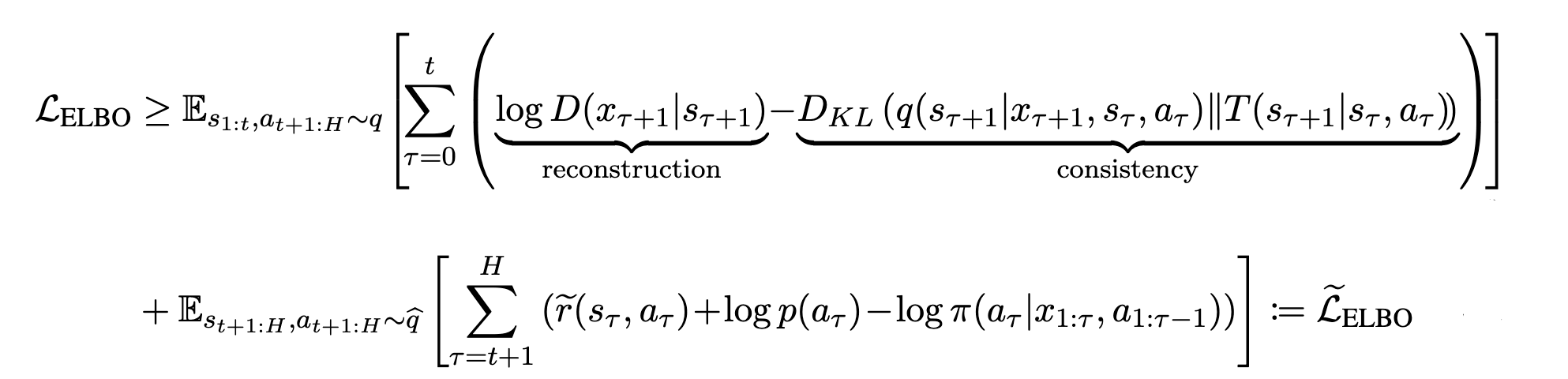Again, it is important to note that this $\widetilde{\mathcal{L}}_{ELBO}$ is the lower-bound of $\mathcal{L}_{ELBO}$. By using this, we can optimize the latent dynamics model and policy. In the next section, I will show you how to implement the system in practice.

### Regarding the implementation of LOMPO

The figure below illustrates the overall model. The image is mapped onto the latent space through the encoder $E_{\theta}$ and represented as $s_{t}$, and then remapped onto the original image by the decoder $D_{\theta}$. Then, as shown in the ELBO formula in the previous section, the latent dynamics model $\widetilde{T}(s_{t}|s_{t-1}, a_{t-1})$ and the inference, $q(s_{t+1}|x_{t+1}, s_{t}, a_{t})$, must be distributed close to each other. Therefore, these models are optimized with respect to the following objective function.

$$\sum_{\tau=0}^{H-1}\left[\mathbb{E}_{q}\left[\log D\left(x_{\tau+1} \mid s_{\tau+1}\right)\right]-\mathbb{E}_{q} D_{K L}\left(q\left(s_{\tau+1} \mid x_{\tau+1}, s_{\tau}, a_{\tau}\right) \| \widehat{T}_{\tau}\left(s_{\tau+1} \mid s_{\tau}, a_{\tau}\right)\right)\right]$$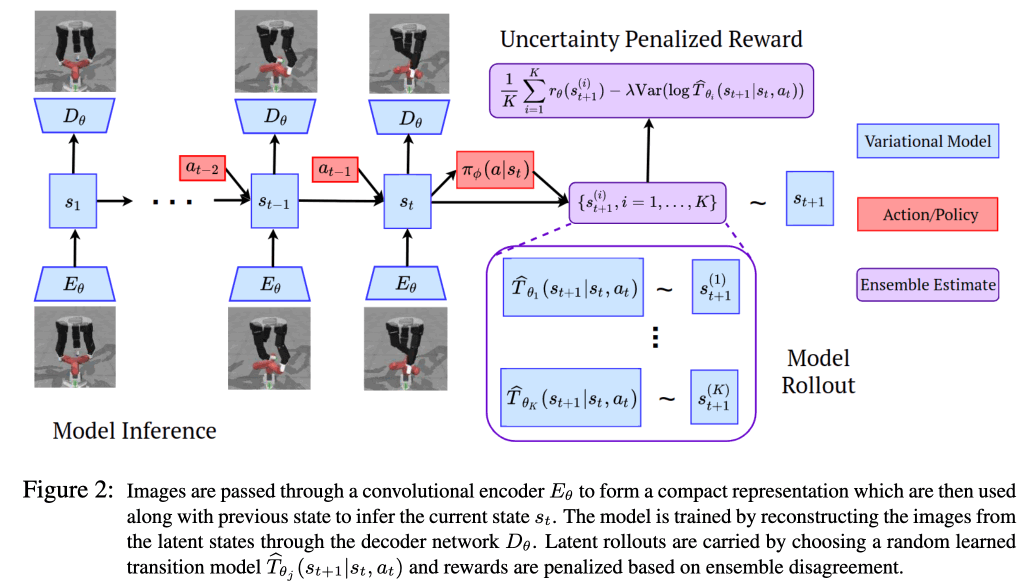The important thing here is to learn an ensemble of latent transition models, which are transition models in the latent space, in order to obtain uncertainty in uncertainty-penalized POMDPs. Suppose we have K models $\{\widetilde{T}_{1}... \widetilde{T}_{K}\}$ are to be learned, uncertainty $u(s_{t}, a_{t})$ is defined as $u(s_{t}, a_{t})=Var(\widetilde{T}_{\theta_{i}}(s_{t}|s_{t-1}, a_{t-1 })\})$ ($i=\{1,... ,K\}$). When optimizing the above objective function, the set of latent dynamic models is trained by randomly sampling from $\{\widetilde{T}_{1}...\widetilde{T}_{K}\}$.

Next, we are concerned with the optimization of policy. In this paper, we learn policy $\pi_{\phi}(a_{t}|s_{t})$ and critic $Q_{\phi}(s_{t}, a_{t})$ on the latent space. To do this, we prepare two replay buffers $\mathcal{B}_{real}$ and $\mathcal{B}_{sample}$. The $\mathcal{B}_{real}$ contains $s_{t}, a_{t}, r, s_{t+1}$ obtained from the real data, and the state uses the real data set $x_{1:H}$ to calculate $s_{1:H} \sim q(s_{1:H}|x_{1:H}, a_{1: H-1})$, which is obtained as follows. On the other hand, in $\mathcal{B}_{sample}$. It contains the data obtained by the ensemble of trained foward models. The reward for doing so is expressed in

$$\widetilde{r}_{t}\left(s_{t}, a_{t}\right)=\frac{1}{K} sum_{i=1}^{K} r_{\theta}\left(s_{t}^{(i)}, a_{t}\right)-\lambda u\left(s_{t}, a_{t}\right)$$.with the addition of the sanction of UNCONFIRMABILITY.

## experiment

The experiments in this paper are designed to answer the following four questions.

- Whether LOMPO is able to solve tasks in a complex dynamics environment
- Performance improvement when comparing LOMPO to existing methods
- Impact of data set quality and size.
-. Can LOMPO be used against real robotic environments?

In order to obtain answers to these questions, we conducted experiments in the following mainly robot-based tasks in the paper.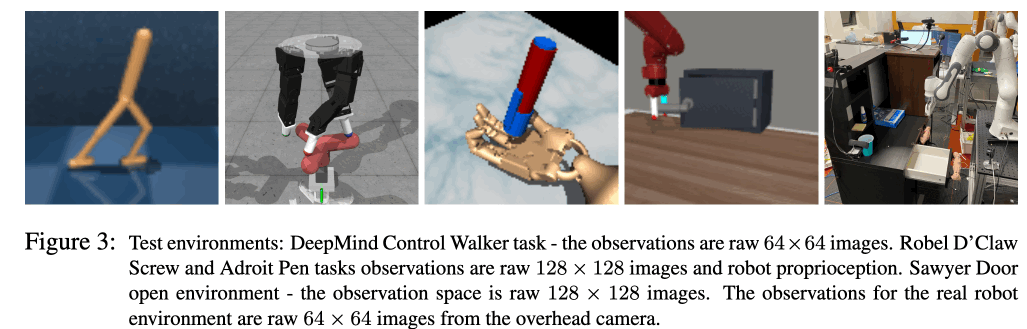### Simulation results

We used Behavior Cloning (BC), Conservative Q-learning (CQL), Offline SLAC, and LMBRL as comparison methods for LOMPO. The results are shown in the table below. In the table below, medium-replay is a test to see if it is possible to learn a policy from an incomplete dataset when collecting offline data until the policy reaches half of the performance of expert policy. Medium-expert is a dataset that contains suboptimal data in the latter part of the replay buffer of the policy learned during data collection. Finally, the expert dataset represents the data sampled from the expert policy, but with a very narrow data distribution. The results in the table below show that the proposed method, LOMPO, basically performs well. However, for the Door Open task, BC shows a better performance, which is due to the narrow data distribution. In the case of the expert data set, the In contrast, D'Claw's performance is better than BC's on the Door Open task, suggesting that it fails to learn the dynamics model on expert datasets with narrow data distributions. On the other hand, the fact that the expert dataset performs better on the D'Claw Screw and Adroit Pen tasks suggests that the task is simpler than that of the Door Open task, and thus the training is more successful.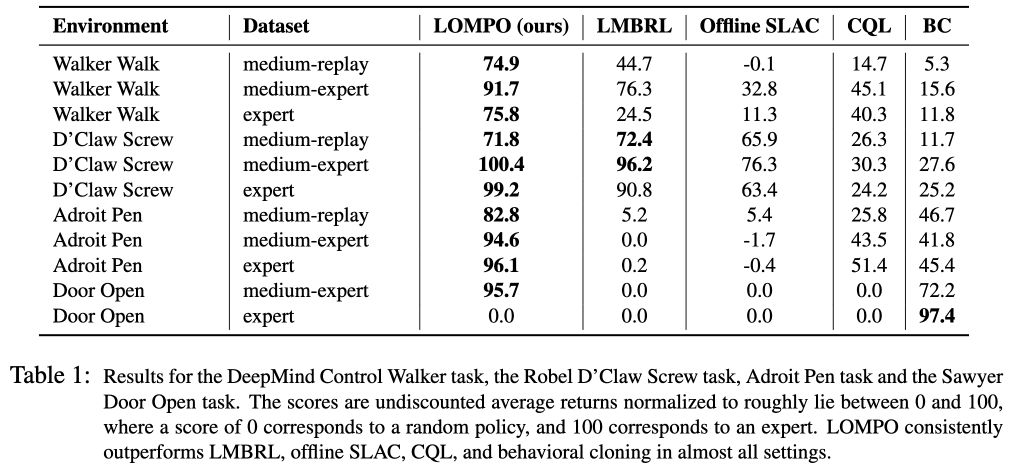### Experiments with real-world robots

In this experiment, we use a real robot to evaluate LOMPO. The robot used is Franka Emika Panda robot arm. As shown in the figure below, only LOMPO successfully learns and solves the task. In contrast, all other comparison methods failed to solve the task.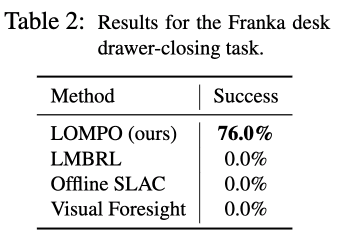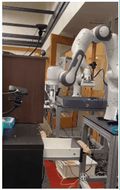## summary

In this article, we introduced LOMPO, a proposed method that enables Offline Model-based RL to learn from image input. Offline RL is a field that is attracting a lot of attention now, and it is very important to be able to learn from images when applying it to robots in the real world . It is expected to be able to learn more complex tasks in the future.